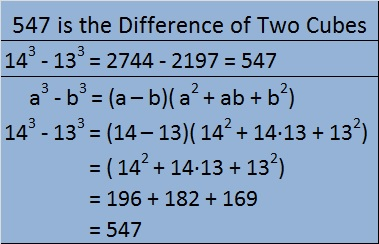# 547 and Level 4

547 is the difference of two consecutive numbers that have been cubed.

Thus 547 = 14³ – 13³.We can use divisibility tricks that easily show that 547 is not divisible by 2, 3, 5, or 11.

• 547 is odd so it’s not divisible by 2
• 5 + 4 + 7 = 16 which is not divisible by 3, so 547 is not divisible by 3
• The last digit of 547 is not 5 or 0 so it is not divisible by 5
• 5 – 4 + 7 = 8 which is not divisible by 11, so 547 is not divisible by 11

Also because it is the difference of 14^3 and 13^3, and 13 and 14 have no common prime factors, 547 also cannot be evenly divided by 13, 14 or any of their prime factors (2 and 7). Thus 547 is not divisible by 2, 3, 5, 7, 11, or 13. Could 547 possibly be a prime number? We only have to try to divide it by 17, 19, and 23 to know for sure! Scroll down past the puzzle to find out.Print the puzzles or type the solution on this excel file: 10 Factors 2015-07-06

—————————————————————————————————

• 547 is a prime number.
• Prime factorization: 547 is prime.
• The exponent of prime number 547 is 1. Adding 1 to that exponent we get (1 + 1) = 2. Therefore 547 has exactly 2 factors.
• Factors of 547: 1, 547
• Factor pairs: 547 = 1 x 547
• 547 has no square factors that allow its square root to be simplified. √547 ≈ 23.38803How do we know that 547 is a prime number? If 547 were not a prime number, then it would be divisible by at least one prime number less than or equal to √547 ≈ 23.4. Since 547 cannot be divided evenly by 2, 3, 5, 7, 11, 13, 17, 19, or 23, we know that 547 is a prime number.

—————————————————————————————————